# Bohr theorem

on power series

If a power series(a1)

converges in the unit disc and its sum has modulus less than, then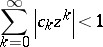(a2)

in the disc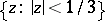. Moreover, the constant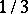cannot be improved.

This formulation of the result of H. Bohr [a1] is due to the work of M. Riesz, I. Schur and F. Wiener.

## Multi-dimensional variations.

Denote bythe largest number such that if the series(a3)

converges in the unit poly-disc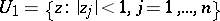and the estimate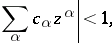(a4)

is valid there, then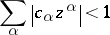(a5)

holds in the homothetic domain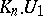; here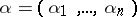, allare non-negative integers,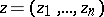,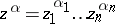.

Regarding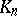, the following is known [a2]: Forone has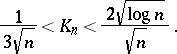(a6)

Next, for the hypercone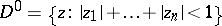, let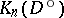be the largest number such that if the series (a3) converges in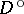and the estimate (a4) is valid there, then (a5) holds in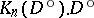.

For the hyperconethe following estimates are true [a3]: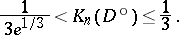Moreover, if, then there exists a series of the form (a3) converging inand such that the estimate (a4) is valid there, but (a5) fails at the point.

Denote by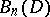the largest numbersuch that if the series (a3) converges in a complete Reinhardt bounded domainand (a4) holds in it, then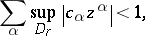(a7)

whereis a homothetic transform of. If, then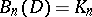. This gives a natural generalization of Bohr's theorem.

The inequality(a8)

is true for any complete bounded Reinhardt domain[a3].

This estimate can be improved for concrete domains [a3]: For the unit hyperconethe following inequality holds: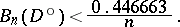(a9)

## Arbitrary bases.

In [a4], Bohr's phenomenon was studied for arbitrary bases in the space of holomorphic functions on an arbitrary domain, by analogy with (a7) (or (a5)). One can easily see that Bohr's phenomenon appears for a given basis only if the basis contains a constant function. It has been proven that if, in addition, all other functions of the basis vanish at some point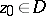, then there exist a neighbourhoodofand a compact subset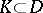such that, whenever a holomorphic function onhas modulus less than, the sum of the maximum in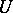of the moduli of the terms of its expansion is less thantoo.

More precisely, one has proven [a4] that ifis a complex manifold andis a basis insatisfying:

i)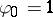;

ii) there exists a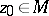such that,, then there exist a neighbourhoodof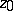and a compact subset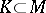such that for all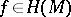,,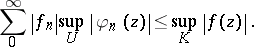For holomorphic functions with positive real part the following assertion (analogous to the initial formulation) holds [a5]. If the function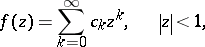has positive real part and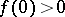, then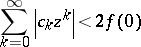in the discand the constantcannot be improved.

Thus, ifis the unit disc and, the Bohr radius in the above assertion and that in the initial assertion are equal. The next results shows that Bohr radii are equal in a more general situation too [a5].

Letbe a complex manifold,and let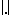be a continuous semi-norm insuch that

a);

b)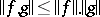. Then the following statements are equivalent:

A)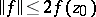iffor all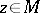and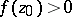;

B)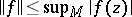for all.

How to Cite This Entry:
Bohr theorem. L. Aizenberg (originator), Encyclopedia of Mathematics. URL: http://encyclopediaofmath.org/index.php?title=Bohr_theorem&oldid=12334
This text originally appeared in Encyclopedia of Mathematics - ISBN 1402006098